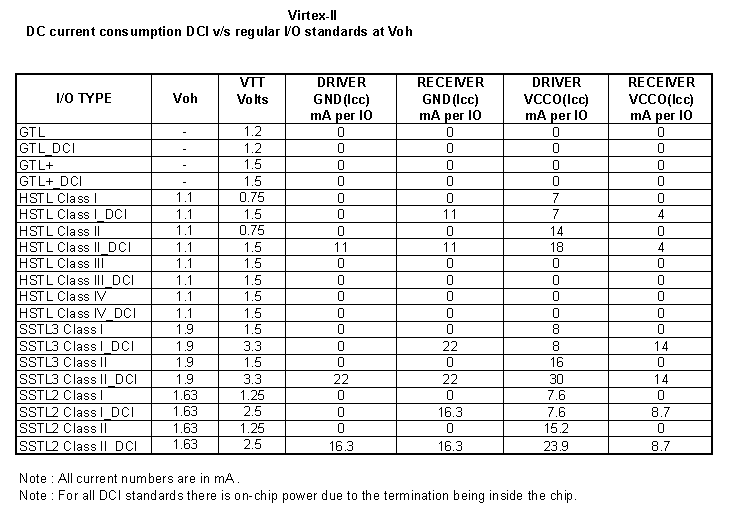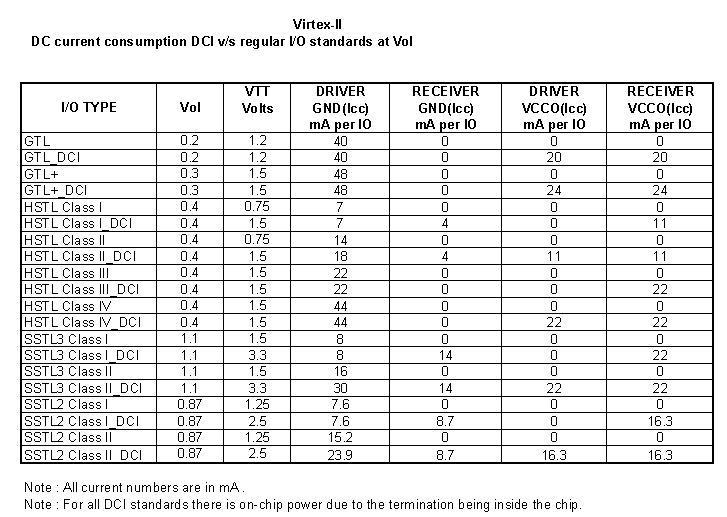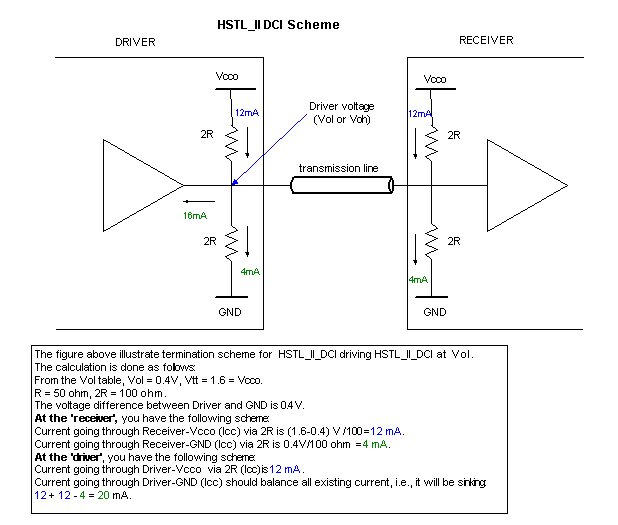# AR# 11661

|

## Description

What is the DC power consumption of DCI I/O?

## Solution

For Virtex-4, Virtex-5 and Spartan-3 Generation of FPGAs, please use the Xilinx Power Estimator (XPE) Spreadsheets to estimate DCI power consumption.

The following is intended as a means to perform analytical power estimates for DCI in Virtex-II devices. The tables below illustrate the non-frequency-dependent current of non-DCI and DCI standards as implemented in Virtex-II devices only:Virtex-II DCI vs. Non-DCI DC current at VOHVirtex-II DCI vs. Non-DCI DC current at VOL

The following figure illustrates the calculation method:Calculation Example for HSTL_II_DCI at VOL

NOTES:

1. These numbers should be used as guidelines for power calculation. The actual VOL/VOH might vary in the board; hence, the actual current might differ from the table above.

2. On both the VOL and VOH tables, the current for SSTL2 and SSTL3 is calculated based on the assumption that the DCI I/O driver will sink or source the same current as the non-DCI termination. In this case, the drivers of SSTL DCI have been weakened to account for the series termination.

For example, the calculation for SSTL3_I at VOL is as follows:

Non-DCI driver:

Current at termination resistor (R) to VCCO at receiver is (Vtt - Vol) / R = (1.5 - 1.1)/ 50 = 8 mA.

(This is also the current sunk in the non-DCI driver to GND.)

DCI driver:

The current sunk by the driver - GND remains the same -- 8 mA.

The current at the receiver termination resistor (2R) to VCCO is (Vcco - Vol)/ 2R = (3.3 - 1.1)/100 = 22 mA.

The balance, the current at receiver termination resistor (2R) to GND, is 22 - 8 = 14 mA.

3. On the VOH table, no current is incurred in HSTL Class III and Class IV. This is because, at VOH, there is no current path to GND.

4. The VRN/P pins will also dissipate power. Because of this and the internal termination, the Virtex-II device will consume more power with XCITE (DCI) than without. Please see (Xilinx Answer 11794) for more information.

5. To see the power consumption during transition and the actual IV data, you must run an IBIS simulation.

AR# 11661
Date 12/15/2012
Status Active
Type General Article
People Also Viewed